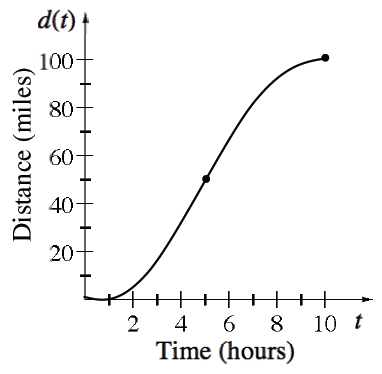### Home > APCALC > Chapter 3 > Lesson 3.3.2 > Problem3-103

3-103.

The graph at right shows the distance a bicyclist travels from Oshkosh to a town $100$ miles away.

1. Describe the velocity of the bicyclist.

The velocity is the slope of a distance graph.

The velocity is always positive. The bicyclist accelerates between $t = 0$ and $t = 5$ and then decelerates between $t = 5$ and $t = 10$. S/he is moving fastest at $t = 5$.

2. What is the bicyclist’s average velocity?

Average velocity between $t = 0$ and $t=10=\frac{\Delta \text{distance}}{\Delta \text{time}}=\frac{d(10)-d(0)}{10-0}$

3. Approximate the bicyclist’s instantaneous velocity at $t = 5$ hours.

IROC at a point $=$ slope of the tangent line at that point.